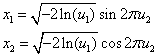ID 772987
Date 12/04/2020
Public

## Special Properties

To improve the efficiency of the algorithms based on the general methods described above, you may have to use special properties of distributions. For example, use of polar coordinates for a pair of independent normal variates enables you to develop an efficient method of random number generation based on 2D inverse transformation known as the Box-Muller method:Generating s-dimensional normally distributed quasi-random sequences with 2D inverse transformation (the VS name is the Box-Muller2 method) is problematic when s is odd, because quasi-random numbers are generated in pairs. One of the options is to generate (s+1)-dimensional normally distributed quasi-random numbers from (s+1)-dimensional quasi-random numbers produced by a basic quasi-random generator and then ignore the last dimension.

Another option is to use the method that produces one normally distributed number from two uniform ones (the VS name is the Box-Muller method). In this case, to generate s-dimensional normally distributed quasi-random numbers, use 2s-dimensional quasi-random numbers produced by a basic quasi-random generator.

For a binomial distribution with parameters m, p, the probability mass function is as follows:For p > 0.5, it is convenient to use the fact thatThus, you can convert a uniform distribution to a general distribution using a number of methods. Two different transformation techniques implemented for the same uniform distribution produce two different sequences of a general distribution, though possessing the same statistical properties.

Consider a simple example. If U1, U2 are two independent random values uniformly distributed over the interval (0, 1), that is, with the distribution function F(x) = x , 0 < x < 1, then the variate X = max(U1, U2) has the distribution F(x) ·F(x). On the one hand, the random number x1 with the maximum distribution from two independent uniform distributions may be derived either from a pair of uniformly distributed random numbers u1, u2 as x1 = max(u1, u2) or from one uniform random number u1 as x1 = sqrt(u1) by applying the inverse transformation method. It is obvious that applying two different methods to one and the same sequence u1, u2, u3, ... gives two absolutely different sequences xi.

You can perform transformations into non-uniform distribution sequences using various methods. The inverse transformation method may be preferable over the acceptance/rejection method for some applications and architectures, while the reverse preference is common for others. Taking this into account, the VS interface provides different options of random number generation for the same probability distribution. For example, a Poisson distribution may be transformed by two different methods: the first, known as PTPE [Schmeiser81], is based on acceptance/rejection and mixture of distributions techniques, while the second one is implemented through transformation of normally distributed random numbers.

The method number calls a method for a specified generator, for example:

viRngPoisson( VSL_METHOD_IPOISSON_PTPE, stream, n, r, lambda )

- calling the PTPE method by passing the method number VSL_METHOD_IPOISSON_PTPE.

viRngPoisson( VSL_METHOD_IPOISSON_POISNORM, stream, n, r, lambda )

- calling transformation from normally distributed random numbers by passing the method number VSL_METHOD_IPOISSON_POISNORM.

For details on methods to be used for specific distributions, see Continuous Distribution Random Number Generators and Discrete Distribution Random Number Generators sections.